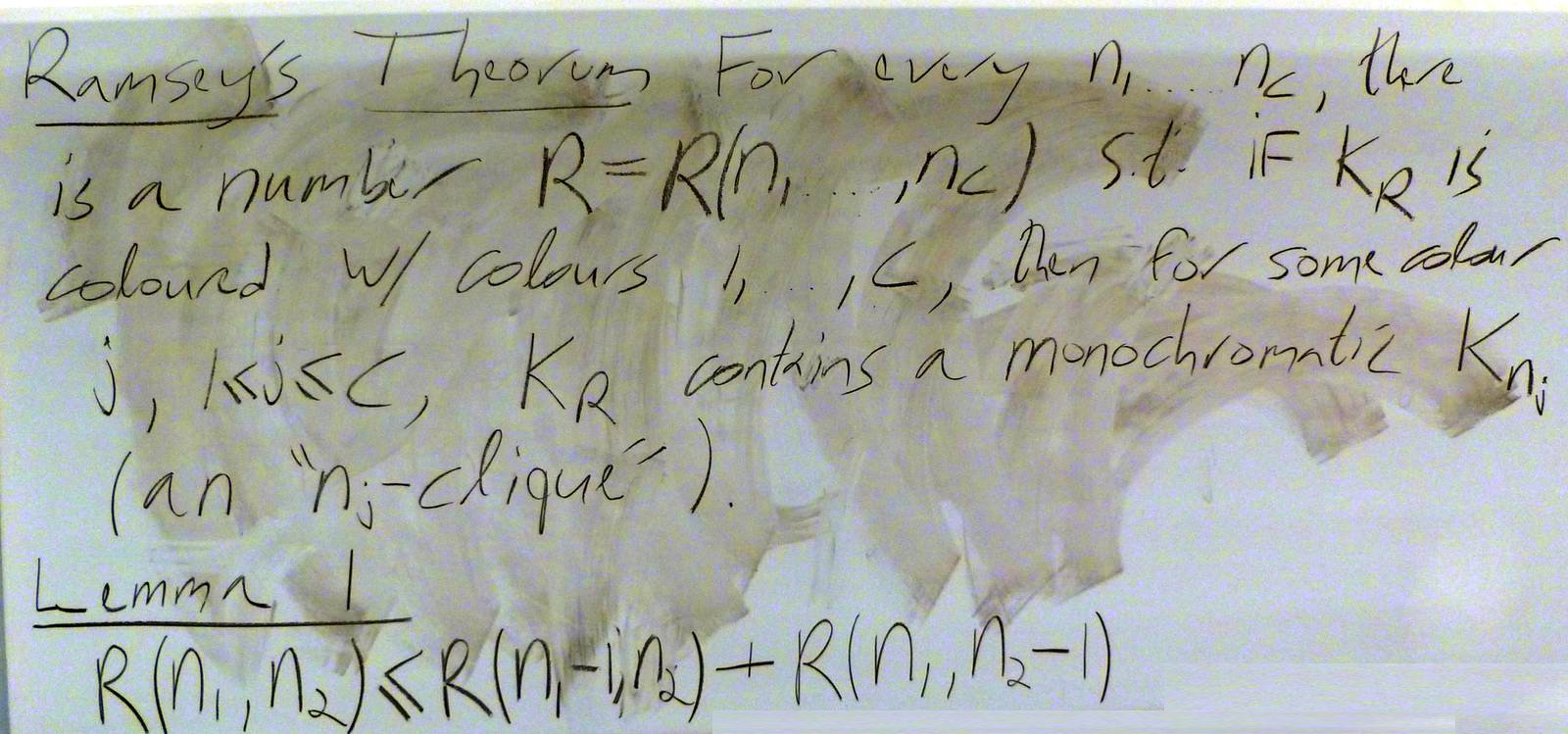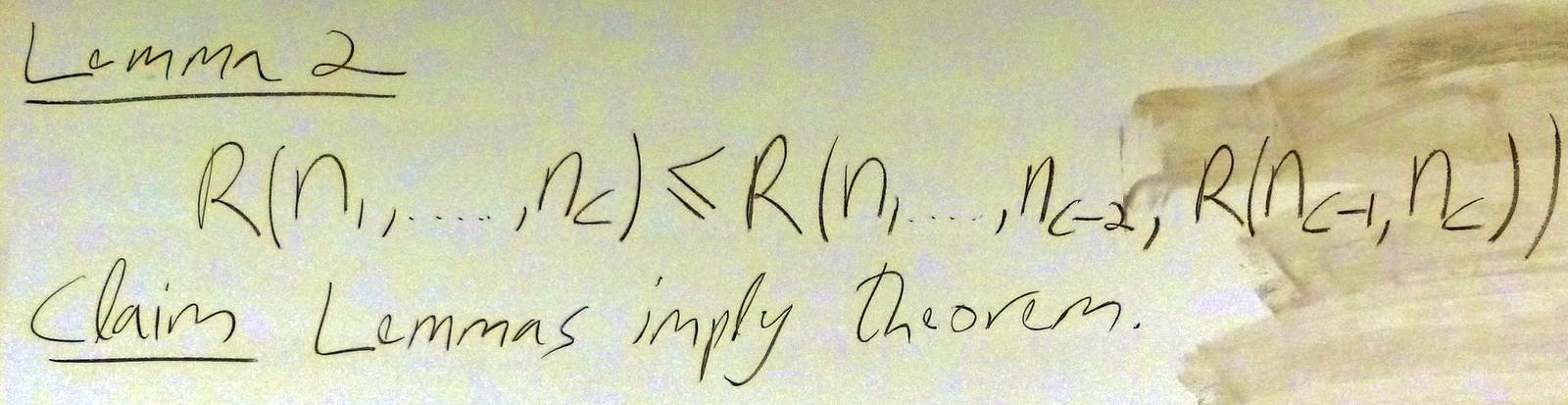$\def\bbN{{\mathbb N}} \def\bbQ{{\mathbb Q}} \def\bbR{{\mathbb R}} \def\bbZ{{\mathbb Z}}$
 © | Dror Bar-Natan: Classes: 2014-15: Math 475 - Problem Solving Seminar: (42) Next: A Mathematica notebook for Problem 10 Previous: A Mathematica notebook for the Ramsey numbers

# Handout for March 12, "Pursue Parity"

Reading. Section 1.10 of Larson's textbook.

Next Quiz. Tuesday March 17 on that section and this handout.

By popular demand - we'll again go over the following:

Problem 0 (Quiz 8 Problem 1.2). Let $\alpha$ be an irrational number. Prove that the set $\{\{n\alpha\}\colon n\in\bbZ\}$ is dense in the unit interval $[0,1]$, where for a real number $x$, $\{x\}$ denotes its "fractional part" - the difference between $x$ and the largest integer $\lfloor x\rfloor$ smaller or equal to $x$.

Problem 1 (Larson's 1.10.1, reworded). Given $9$ distinct points in $\bbZ^3$, show that there is some point in $\bbZ^3$ which is exactly half way between two of these $9$ points.

Problem 2.

1. (Larson's 1.10.2, modified). Is there a closed knight-path on a $7\times 7$ chessboard that visits every square of the board exactly once?
2. (An old classic). Removing two opposite corners of an $8\times 8$ chessboard, can you cover what remains with $31$ domino pieces of size $2\times 1$?
3. (A classic variant). Can you cover an $8\times 8$ chessboard with $21$ rectangles of size $3\times 1$ and a single extra $1\times 1$ square?
4. (A 3D variant.) Can you pack 125 boxes of size $4\times 2\times 1$ inside one $10\times 10\times 10$ cube?

Problem 3 (a classic.)

1. Can you draw a quadrilateral (non-convex!) and an additional straight line, such that the straight line cuts through the interior of each of the quadrilateral's edges?
2. Can you draw a pentagon (non-convex!) and an additional straight line, such that the straight line cuts through the interior of each of the pentagon's edges?

Problem 4 (Larson's 1.10.4, reworded). Let $n$ be an odd integer and let $A$ be a symmetric $n\times n$ "Latin" matrix - every row and every column in $A$ is a permutation of $\{1,2,\ldots,n\}$. Show that the diagonal of $A$ is also a permutation of $\{1,2,\ldots,n\}$.

Problem 5 (a classic.) 10 prisoners are made to stand in a line by an evil warden, who also places either a black hat or a white hat on the head of each prisoner. Each prisoner can see all the hats in front of her, but not her own hat or the hats behind her. The evil warden than asks each prisoner for the colour of her hat, starting from the last one who sees all but herself and ending with the first one who sees no one. The prisoners are allowed to make at most one mistake; if they make more than one mistake, they are all executed. If not, they are allowed to participate in the game of Problem 5. Assuming the prisoners know in advance that they will be subject to this game, can they devise a strategy that will allow them to survive at least until the next problem?

Problem 6 (a vicious classic, not for marks.) Our evil warden makes infinitely many prisoners stand in a circle so that each one can see the colour of the hats (black or white) on all heads but herself. All at once, each has to shout the colour of the hat on her head; if only finitely many get it wrong, they are all freed. But if more than just finitely many get it wrong, well, you know what happens in prisoner problems that involve an evil warden. Assuming they had the day before to devise a strategy, can they survive?

Problem 7 (Larson's 1.10.8, reworded). Let $n$ be odd and $\sigma$ a permutation of $\{1,2,\ldots,n\}$. Prove that the product $(\sigma_1-1)(\sigma_2-2)\cdots(\sigma_n-n)$ is even.
(And while you're at it, here's a kids' problem. Simplify the expression $(a-t)(b-t)(c-t)\cdots(y-t)(z-t)$ as best as you can).

Problem 8 (Larson's 1.10.10, reworded). Show that for every positive integer $a$, the equation $x^2-y^2=a^3$ has solutions with $x,y\in\bbZ$.

Problem 9 (from the $x+y$ movie trailer). $n$ playing cards are placed in a sequence in front of you, some face up and some face down. At any step, you can flip a face up card and any number of cards on its right, no matter which face they are showing. Show that after finitely many steps you will necessarily be facing a sequence of face-down cards.

Problem 10 (Larson's 3.3.20, reworded). Prove that if $n>1$, the sum $1+\frac12+\frac13+\ldots+\frac1n$ is not an integer.
Hint. Multiply by $\operatorname{lcm}(1,2,\ldots,n)$ and consider the parity of the result.

Problem 11 (not for marks). Complete your understanding of Ramsey's theorem!